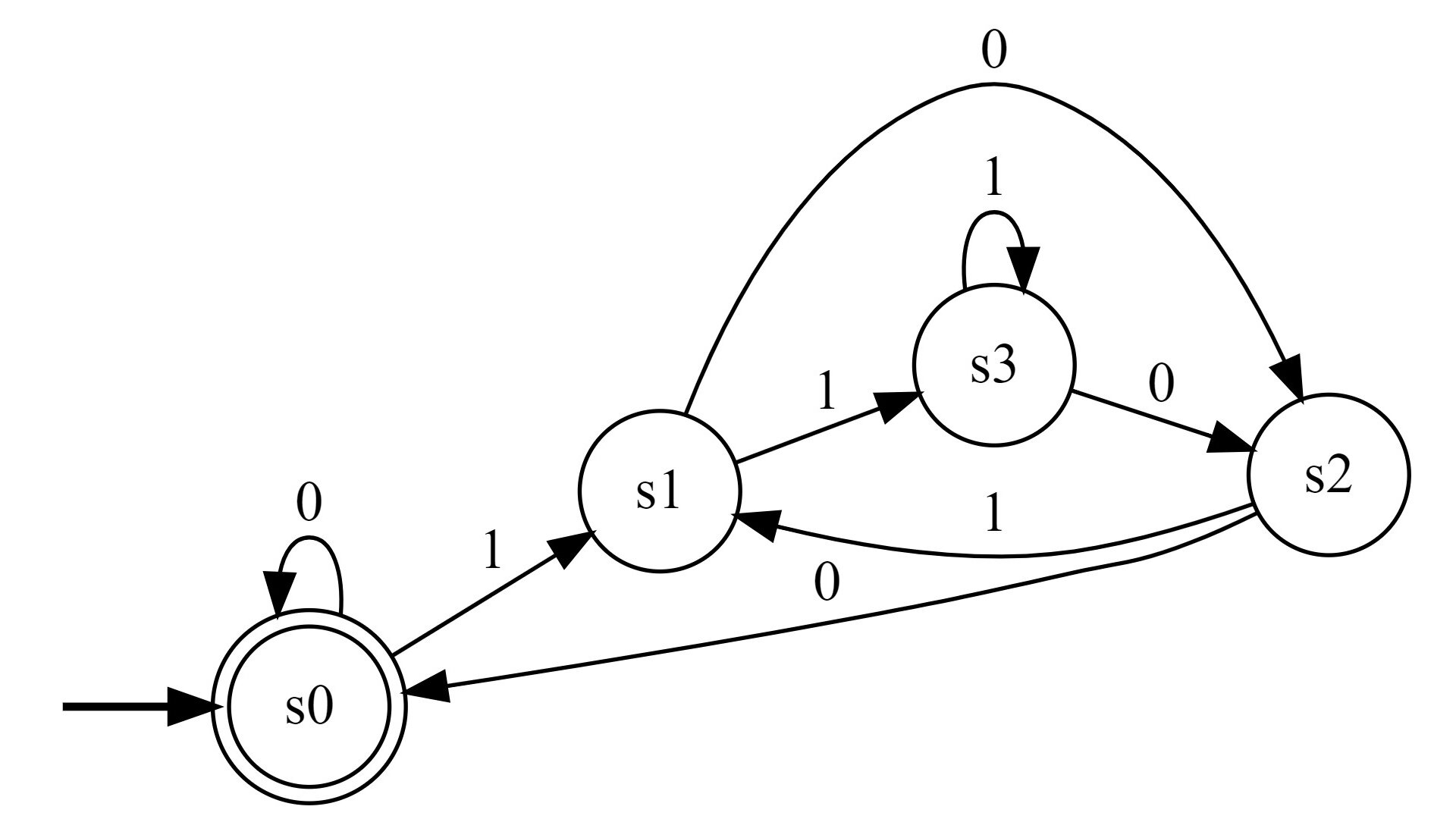# Numbers in congruence classes are regular languagesIn this post we will consider natural Radix-b numbers in positional number systems. The congruence class of any such arbitrary natural number can be determined by a finite automata, and thus, intuitively speaking, the language of all Radix-b numbers that satisfy some fixed properties modulo b is regular.

## Radix-b numbers divisible by m

The key idea behind the automation construction is that after reading a digit, the new congruence class depends only on the current read digit and on the previous congruence class. There are only $m < \infty$ congruence classes so we can construct a finite automata that accepts all numbers divisible by m: \begin{aligned} A &= (Z,\Sigma,\delta, z_0, E) \\ Z &:= \{0,\dots, m-1\} \\ \Sigma &:= \{0,\dots, b-1\} \\ E &:= \{0\} \\ z_0 &:= 0 \\ \delta(r,d) &:= r \cdot b + d \bmod{m} \end{aligned}

The correctness of the transition function can be seen as follows: when the automation is in state $r$, the number that has been read is in the congruence class $r$ modulo $m$. So we can write it as $mk+r$. By multiplying with $b$ (i.e. shift the number by 1 digit to the left) and adding $d$ we get the new congruence class: \begin{aligned} \delta(r,d) &= (mk + r)\cdot b + d \bmod{m} \\ &= mkb + rb + d \bmod{m} \\ &= rb + d \bmod{m} \end{aligned}

Of course, by altering the set of final states $E$ we can accept not only multiples of $m$, but any such number $n$ with $n \bmod{m} \in E$ for any $E \subseteq Z$.

## Binary numbers modulo 4

For example, we can construct a finite automation that accepts all binary ($b = 2$) numbers divisible by 4 ($m = 4$):The transitions are obtained as follows: \begin{aligned} 0 \cdot 2 \bmod{4} = 0 &\implies \delta(0, 0) = 0, \delta(0, 1) = 1 \\ 1 \cdot 2 \bmod{4} = 2 &\implies \delta(1, 0) = 2, \delta(1, 1) = 3 \\ 2 \cdot 2 \bmod{4} = 0 &\implies \delta(2, 0) = 0, \delta(2, 1) = 1 \\ 3 \cdot 2 \bmod{4} = 2 &\implies \delta(3, 0) = 2, \delta(3, 1) = 3 \end{aligned}

In this particular case the constructed automation is not minimal. States s1 and s3 are equivalent (this can be formally proven with the Myhill-Nerode theorem) and can be replaced by one state:As languages accepted by DFA’s are exactly the regular languages, we can transform any DFA in a regular expression to see the exact structure of numbers divisible, for example, by 4. Unfortunately such regular expressions are very long when constructed with the Kleene or with the Arden method by a computer. These regular expressions must be massively simplified in order to be readable. With the Kleene construction I’ve implemented in this project we get the following regular expression:

ε|0|(ε|0)0*(ε|0)|(1|(ε|0)0*1)(1|0*1)*0*(ε|0)|(1|(ε|0)0*1)(1|0*1)*0((1|00*1)(1|0*1)*0)*(0|00*(ε|0)|(1|00*1)(1|0*1)*0*(ε|0))


It can be simplified by computer heuristics down to:

ε|0|1(1|01)*00|(0|ε|1(1|01)*00)(0|1(1|01)*00)*(0|ε|1(1|01)*00)


Still, the regular expression is not so readable. By transforming it further by hand, we can prove that it is equivalent to:

ε|0|1(1|01)*00|(0|ε|1(1|01)*00)(0|1(1|01)*00)*(0|ε|1(1|01)*00) =
ε|0|1(1|01)*00|(ε|0|1(1|01)*00)(0|1(1|01)*00)*(ε|0|1(1|01)*00) =
ε|0|1(1|01)*00|(0|1(1|01)*00)*(ε|0|1(1|01)*00) =
ε|0|1(1|01)*00|(0|1(1|01)*00)* =
ε|0|(0|1(1|01)*00)* =
ε|0|(0|1(1|0)*00)* =
ε|0|0*(1(1|0)*000*)* =
ε|0|0*(1(1|0)*0*00)* =
ε|0|0*(1(1|0)*00)* =
ε|0|0*(1(0|1)*00)* =
ε|0|0*(1(0|1)*00|ε) =
ε|0|0*|0*1(0|1)*00 =
ε|0|0*|0*(0|1)*00 =
ε|0|0*(0|1)*00 =
ε|0|(0|1)*00


So, any binary number divisible by 4 must be zero or end with 00.

## Other examples

### Decimal numbers divisible by 20### Decimal numbers divisible by 75### Hexadecimal numbers divisible by 24